# Heat Sinks: A Step-by-Step Guide to Getting the Heat Out of Your Design

By Aaron Yarnell

Heat sinks are important! A significant aspect of circuit design, they deliver an effective route for heat to be transferred out and away from electronic devices – think BJTs, MOSFETs, and linear regulators – and into ambient air.

Their role is in creating a larger surface area on a heat-producing device, and in doing so, allowing for a more efficient transfer of heat out and into its surroundings. An improved thermal path out of the device lowers any temperature rise at the junction of the component.

This article aims to present an advanced introduction on the issue of choosing a heat sink, drawing on the thermal data from the device application, together with the specifications supplied by the heat sink provider.

## Is a heat sink needed?

For the purpose of this article, we will suppose that the application in question has a transistor housed in a TO-220 package, where conduction and switching losses equal a power dissipation of 2.78 W. In addition, the ambient operating temperature will not go above 50°C. Is a heat sink necessary for this transistor?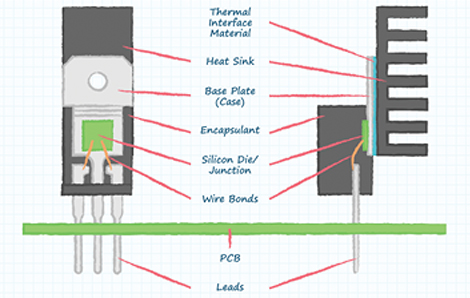Figure 1: Front and side view of a typical TO-220 package with a heat sink (Image source: CUI Inc.)

Firstly, one must assemble and digest the features of all the thermal impedances that are likely to prevent the 2.78 W from being dissipated away into ambient air. If they cannot be dispersed efficiently then the junction temperature within the TO-220 package will increase above the maximum desired operating temperature, which, for this application we will establish to be 125°C.

In general, transistor suppliers record any junction-to-ambient thermal impedance, shown with the symbol Rθ J-A and measured in units of °C/W. The unit signifies the amount the junction temperature is expected to increase above the ambient temperature around the TO-220 package for each unit of power (Watt) dissipated within the device.

To put this into context, when a transistor supplier documents that the thermal impedance from junction-to-ambient is 62°C/W, the 2.78 W of dissipation inside the TO-220 package will make the junction temperature increase to 172°C above the ambient temperature; calculated as 2.78 W x 62°C/W. If the worst-case ambient temperature for this device is assumed to be 50°C, then the junction temperature will reach 222°C, calculated as 50°C + 172°C. Since this far outstrips the specified maximum silicon temperature of 125°C, a heat sink is definitely required.

Connecting a heat sink to the application will significantly reduce the junction-to-ambient thermal impedance. At the next stage, decide how low the thermal impedance pathway is required to be to deliver a safe and reliable operation.

## Establishing thermal impedance paths

In order to determine the thermal impedance pathway, begin with the greatest tolerable rise in temperature. If the largest ambient operating temperature of the device is 50°C, and we have already established that the silicon junction needs to stay at 125°C or less, then the highest permissible temperature rise sits at 75°C; that’s calculated as 125°C - 50°C.

The next step is to calculate the greatest tolerable thermal impedance between the junction itself and the ambient air. If the highest permissible temperature increase stands at 75°C and the Watts dissipated within the TO-220 package are measured at 2.78 W, then the greatest permissible thermal impedance is 27°C/W; calculated as 75°C ÷ 2.78 W.

Lastly, count up all of the thermal impedance pathways, from silicon junction to ambient air, and confirm that their sum is less than the largest permissible thermal impedance; which is 27°C/W as calculated above.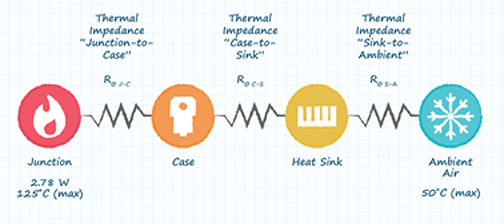Figure 2: Graphic illustration of the thermal impedances that should be calculated and added between the junction and the ambient air in a typical TO-220 application. (Image source: CUI Inc.)

In the Figure 2 graphic, it can be seen that the first thermal impedance needed is the ‘junction-to-case’, represented with the symbol Rθ J-C. This denotes how easy it is for heat to be transferred from the junction where heat is generated, and out to the device’s surface, denoted as TO-220 in this example. Generally, suppliers’ datasheets will list this impedance, together with the junction-to-ambient value. Here, the assumed junction-to-case thermal impedance is rated at 0.5°C/W.

Represented by the symbol Rθ C-S, the second thermal impedance needed is the ‘case-to-sink’, a gauge of how easily heat can be transferred from the device’s outside case to the surface of the heat sink. Since there are sometimes irregularities in the two surfaces, it is normally recommended to apply a thermal interface material – TIM or ‘thermal compound’ – between the surfaces of the TO-220 case and the heat sink base to make sure, from a thermal standpoint, that they are both fully engaged. The application of a TIM will significantly improve the transfer of heat from the TO-220’s surface to the heat sink, although its associated thermal impedance must be considered.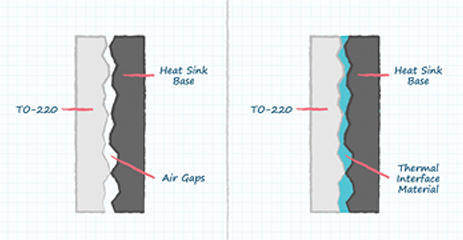Figure 3: Magnified surface-to-surface illustration depicting the need for a Thermal Interface Material (TIM) (Image source: CUI Inc.)

## Thermal interface materials explained

Generally speaking, TIMs are characterized by their thermal conductivity, in units of measurement by Watts per Meters-Celsius (W/(m °C)) or Watts per Meters-Kelvin (W/(m K)). In this example, Celsius and Kelvin are transposable as both use the same increase of temperature measurement, where the rise and fall of temperature are being calculated; for example, a rise in temperature of 45°C is the same as a 45 K temperature rise.

The unit of meters is included due to the fact that the TIM’s impedance relies upon the ratio of thickness (TIM thickness in meters) over the whole area (the area over which the TIM covers in meters2), resulting in 1/m (calculated as m/m2 = 1/m). In this  example, a thin layer of TIM is applied over the metal tab area of TO-220’s case surface, with specific properties and application details as follows: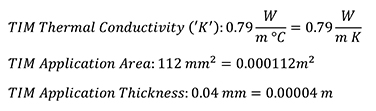Using the properties listed above, the thermal impedance of the TIM can be calculated with the following equation, using meters for consistency: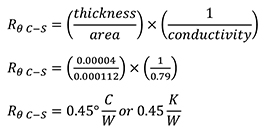## Choosing a heat sink

The last thermal impedance needed is the ‘sink-to-ambient’, which is represented by the symbol Rθ S-A. This calculation reveals how easily heat can be transferred from the base of the heat sink out to surrounding ambient air. Electronic components manufacturer CUI is a heat sink supplier who provides graphs like the one pictured in Figure 4 to demonstrate how easily heat can be transferred from the heat sink to ambient air via different airflow loads and conditions.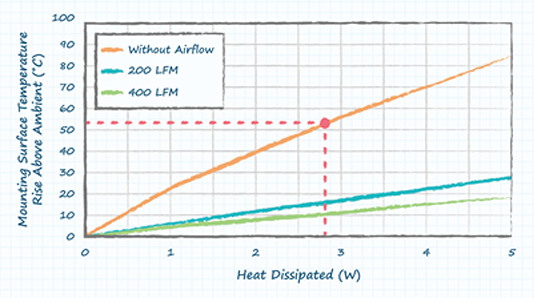Figure 4: Graph showing the typical heat sink mounting surface temperature rise above ambient (Image source: CUI Inc.)

In this example, it is assumed that the device operates under natural convection conditions without any airflow. The graph can be used to calculate the final thermal impedance, sink-to-ambient, for this specific heat sink. The amount the surface temperature rises above ambient, divided by the heat dissipated, gives a result for thermal impedance at that specific operating condition. Here, the dispersed heat is 2.78 W, resulting in a surface temperature rise above ambient of 53°C. Dividing 53°C by 2.78 W will result in a sink-to-ambient thermal impedance of 19.1°C/W.

In previous calculations, the maximum impedance permitted between the junction and ambient air stood at 27°C/W. Subtracting the impedance of the junction-to-case (0.5°C/W) and the impedance of the case-to-sink (0.45°C/W) results in the maximum allowance for the heat sink, calculated as 26.05°C/W; worked out as 27°C/W - 0.5°C/W - 0.45°C/W.

For the purposes of this example, under these assumed conditions, a thermal impedance of 19.1°C/W for this heat sink falls well below the previous calculation of an allowance of 26.05°C/W. This converts to a cooler silicon junction temperature inside the TO-220 package as well as a wider thermal margin within the design. In addition, the maximum temperature of the junction can be approximated by adding together all of the thermal impedances, then multiplying them by the number of Watts dissipated at the junction, and, finally, adding the result to the maximum ambient temperature, as follows:The example demonstrated here reveals just how important heat sinks are in the thermal management of an application. If the heat sink had been omitted, the silicon junction within the TO-220 package would have exceeded the design’s rated limit of 125°C by a wide margin. The process used here can simply be modified and repeated in order to help designers select the right heat sinks that are properly sized for a range of different applications.

## Conclusion

Heat sinks have a significant role to play in circuit design due to the fact that they deliver an efficient pathway for heat to be transferred into ambient air and away from electronic devices. By identifying the maximum temperature of the ambient environment together with the power dissipated within the device,  optimization of the heat sink selection can be obtained; neither too small to cause burnout, nor too large to waste money. In addition, consider the important role that TIMs play in transferring heat efficiently and consistently between two surfaces.

Lastly, once the parameters of the application have been defined – the ambient temperature, power dissipation, and thermal impedance paths – review CUI’s portfolio of board-level heat sinks to identify the suitable model for the project’s cooling needs.

Disclaimer: The opinions, beliefs, and viewpoints expressed by the various authors and/or forum participants on this website do not necessarily reflect the opinions, beliefs, and viewpoints of Digi-Key Electronics or official policies of Digi-Key Electronics.

### About this author

Aaron Yarnell

Article provided by Aaron Yarnell, Field Applications Engineering Manager, CUI.